## 14.4.3 RDT-Based Methods

The rapidly exploring dense tree (RDT) family of methods, which includes the RRT, avoids maintaining a lattice altogether. RDTs were originally developed for handling differential constraints, even though most of their practical application has been to the Piano Mover's Problem. This section extends the ideas of Section 5.5 fromtoand incorporates differential constraints. The methods covered so far in Section 14.4 produce approximately optimal solutions if the graph is searched using dynamic programming and the resolution is high enough. By contrast, RDTs are aimed at returning only feasible trajectories, even as the resolution improves. They are often successful at producing a solution trajectory with relatively less sampling. This performance gain is enabled in part by the lack of concern for optimality.

Let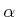denote an infinite, dense sequence of samples in. Let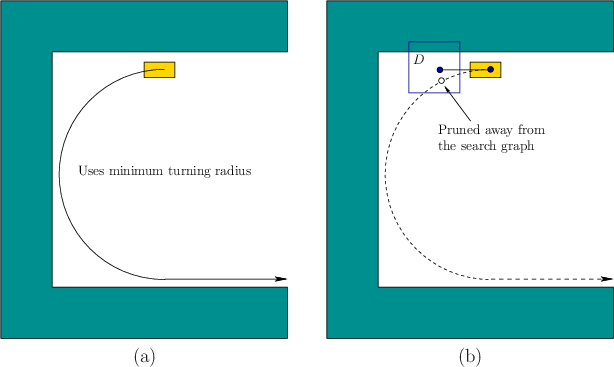denote a distance function on, which may or may not be a proper metric. The distance function may not be symmetric, in which case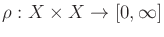represents the directed distance from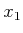to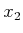.

The RDT is a search graph as considered so far in this section and can hence be interpreted as a subgraph of the reachability graph under some discretization model. For simplicity, first assume that the discrete-time model of Section 14.2.2 is used, which leads to a finite action set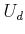and a fixed time interval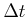. The set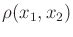of motion primitives is all action trajectories for which some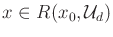is held constant from time 0 to. The more general case will be handled at the end of this section.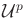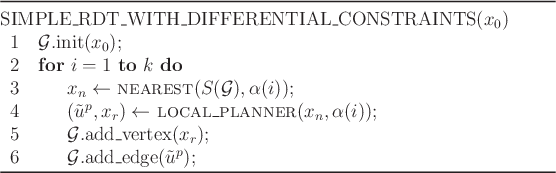Paralleling Section 5.5.1, the RDT will first be defined in the absence of obstacles. Hence, let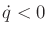. The construction algorithm is defined in Figure 14.19; it may be helpful to compare it to Figure 5.16, which was introduced onfor the Piano Mover's Problem. The RDT, denoted by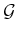, is initialized with a single vertex at some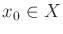. In each iteration, a new edge and vertex are added to. Line 3 uses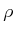to choose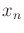, which is the nearest point to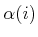in the swath of. In the RDT algorithm of Section 5.5, each sample ofbecomes a vertex. Due to the BVP and the particular motion primitives in, it may be difficult or impossible to precisely reach. Therefore, line 4 calls an LPM to determine a primitive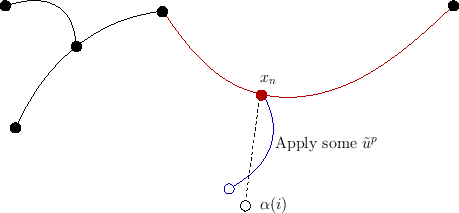that produces a new state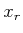upon integration from. The result is depicted in Figure 14.20. For the default case in whichrepresents the discrete-time model, the action is chosen by applying all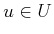over timeand selecting the one that minimizes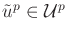. One additional constraint is that ifhas been chosen in a previous iteration, then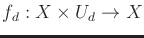must be a motion primitive that has not been previously tried from; otherwise, duplicate edges would result inor time would be wasted performing collision checking for reachability graph edges that are already known to be in collision. The remaining steps add the new vertex and edge from. Ifis contained in the trajectory produced by an edge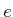, thenis split as described in Section 5.5.1.

Subsections
Steven M LaValle 2012-04-20